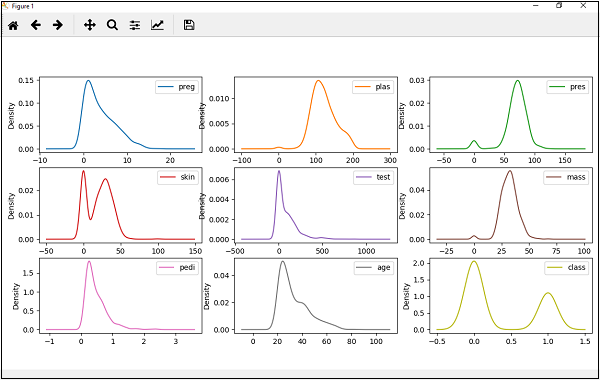# Machine Learning with Python - Density Plots

Another quick and easy technique for getting each attributes distribution is Density plots. It is also like histogram but having a smooth curve drawn through the top of each bin. We can call them as abstracted histograms.

## Example

In the following example, Python script will generate Density Plots for the distribution of attributes of Pima Indian Diabetes dataset.

from matplotlib import pyplot
path = r"C:\pima-indians-diabetes.csv"
names = ['preg', 'plas', 'pres', 'skin', 'test', 'mass', 'pedi', 'age', 'class']
data.plot(kind='density', subplots=True, layout=(3,3), sharex=False)
pyplot.show()


## OutputFrom the above output, the difference between Density plots and Histograms can be easily understood.

machine_learning_with_python_understanding_data_with_visualization.htm